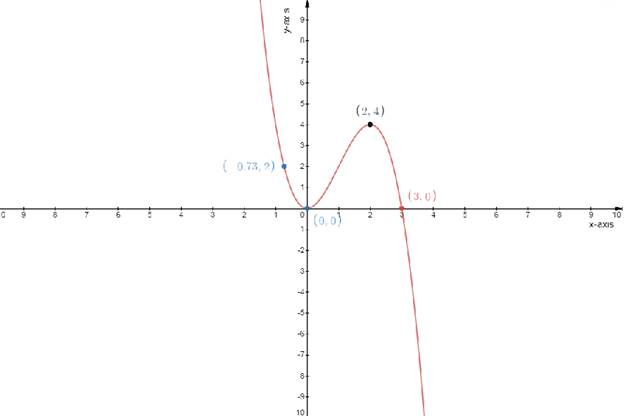# The differential equation of the given equation### Single Variable Calculus: Concepts...

4th Edition
James Stewart
Publisher: Cengage Learning
ISBN: 9781337687805### Single Variable Calculus: Concepts...

4th Edition
James Stewart
Publisher: Cengage Learning
ISBN: 9781337687805

#### Solutions

Chapter 3.1, Problem 37E
To determine

## To find:The differential equation of the given equation

Expert Solution

The equation is f(1)=3

### Explanation of Solution

Given:

The function is

f(x)=3x2x3

Concept used:

Definition of the differentiation:-Differentiation is the action of computing a derivative

The derivative of a function y=f(x) of a variable x is a measure of the rate at which the value y of the function changes with respect to x

Calculation:

The function

f(x)=3x2x3...................(1)

The derivative of a function

y=f(x)y=f(x)=dydx

Differentiating the equation (1) with respect to x

dydx=ddx(3x2x3)dydx=6x3x2f(x)=6x3x2.....................(2)

Replacing x by a in equation (2)

f(x)=6x3x2f(a)=6a3a2

Putting the given value a=1

f(1)=6(1)3(1)2f(1)=63f(1)=3

Draw the table

y=f(x)=3x2x3

Test one point in each of the region formed by the graph

If the point satisfies the function then shade the entire region to denote that every point in the region satisfies the function

 x−axis 0 2 3 0.73 y−axis 0 4 0 2### Have a homework question?

Subscribe to bartleby learn! Ask subject matter experts 30 homework questions each month. Plus, you’ll have access to millions of step-by-step textbook answers!